Visualize two plots on a single screen. The plot at the top is a histogram representing the frequency of the level. The plot at the bottom is a bar chart representing the frequency of the level.

# S3 method for bins
plot(x, typographic = TRUE, base_family = NULL, ...)

## Arguments

x an object of class "bins", usually, a result of a call to binning(). logical. Whether to apply focuses on typographic elements to ggplot2 visualization. The default is TRUE. if TRUE provides a base theme that focuses on typographic elements using hrbrthemes package. character. The name of the base font family to use for the visualization. If not specified, the font defined in dlookr is applied. (See details) arguments to be passed to methods, such as graphical parameters (see par).

## Details

The base_family is selected from "Roboto Condensed", "Liberation Sans Narrow", "NanumSquare", "Noto Sans Korean". If you want to use a different font, use it after loading the Google font with import_google_font().

binning, print.bins, summary.bins.

## Examples

# Generate data for the example
# \donttest{
heartfailure2 <- heartfailure
heartfailure2[sample(seq(NROW(heartfailure2)), 20), "platelets"] <- NA

# Binning the platelets variable. default type argument is "quantile"
bin <- binning(heartfailure2$platelets, nbins = 5) plot(bin)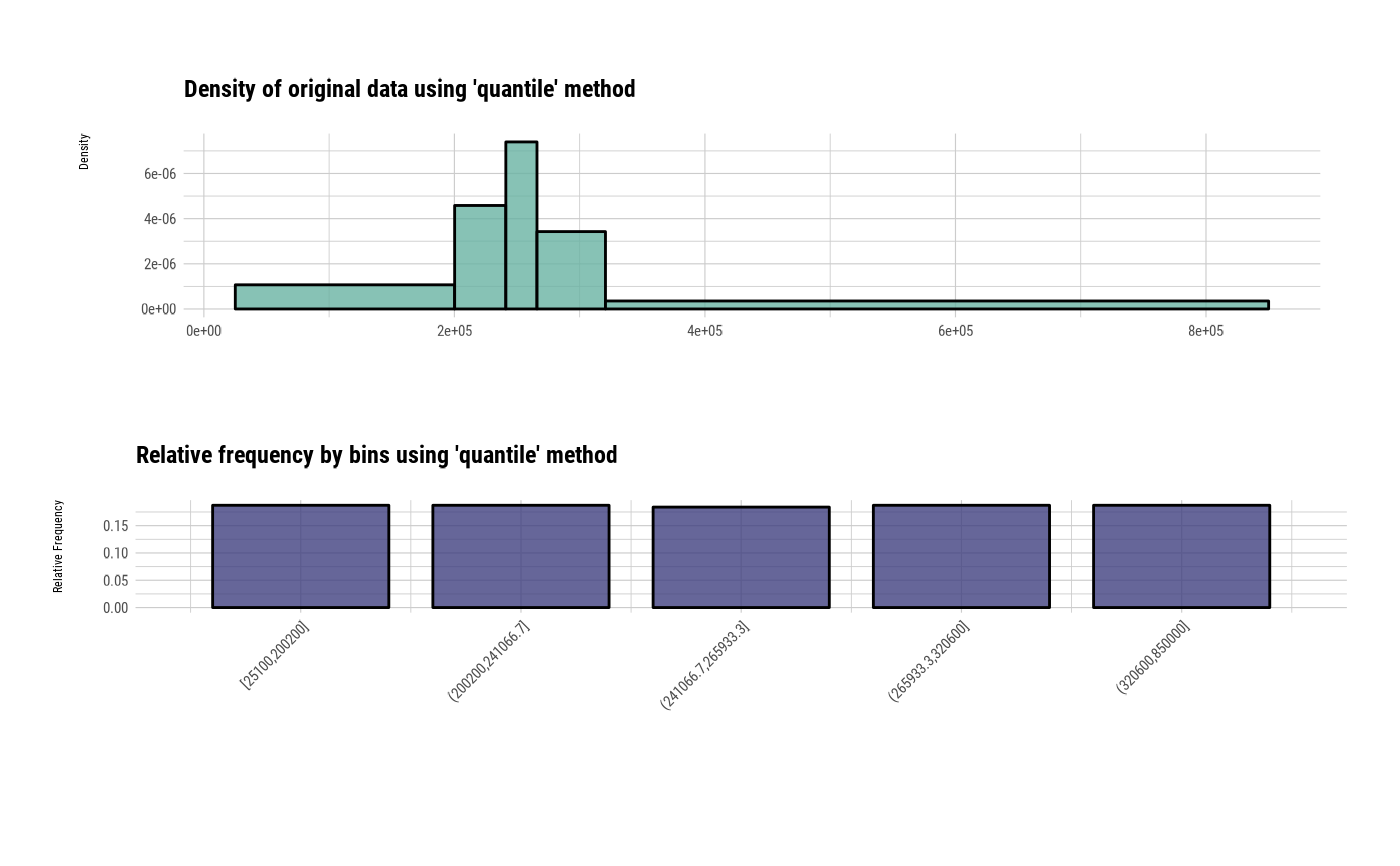# Using another type arguments bin <- binning(heartfailure2$platelets, nbins = 5, type = "equal")
plot(bin)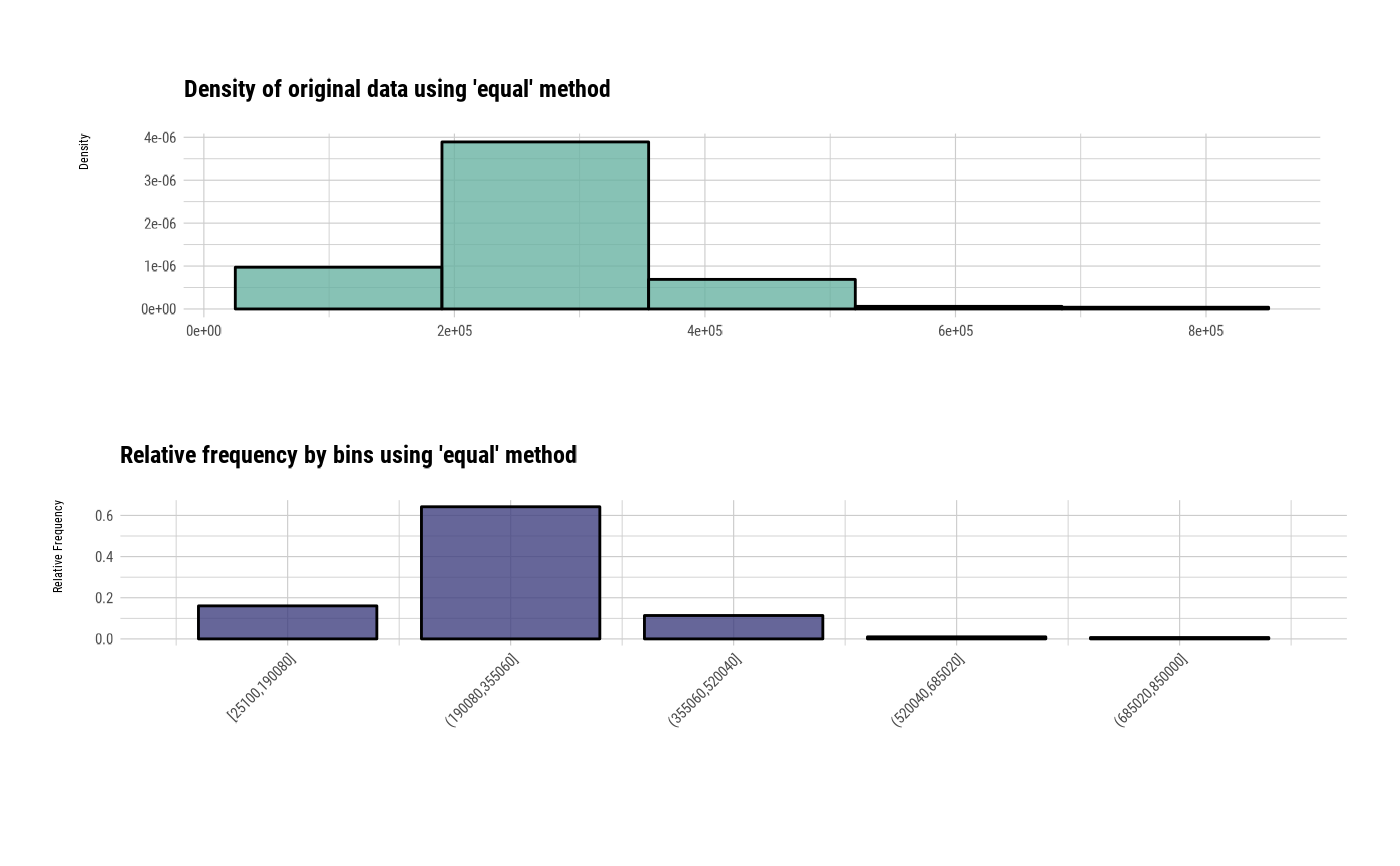bin <- binning(heartfailure2$platelets, nbins = 5, type = "pretty") plot(bin)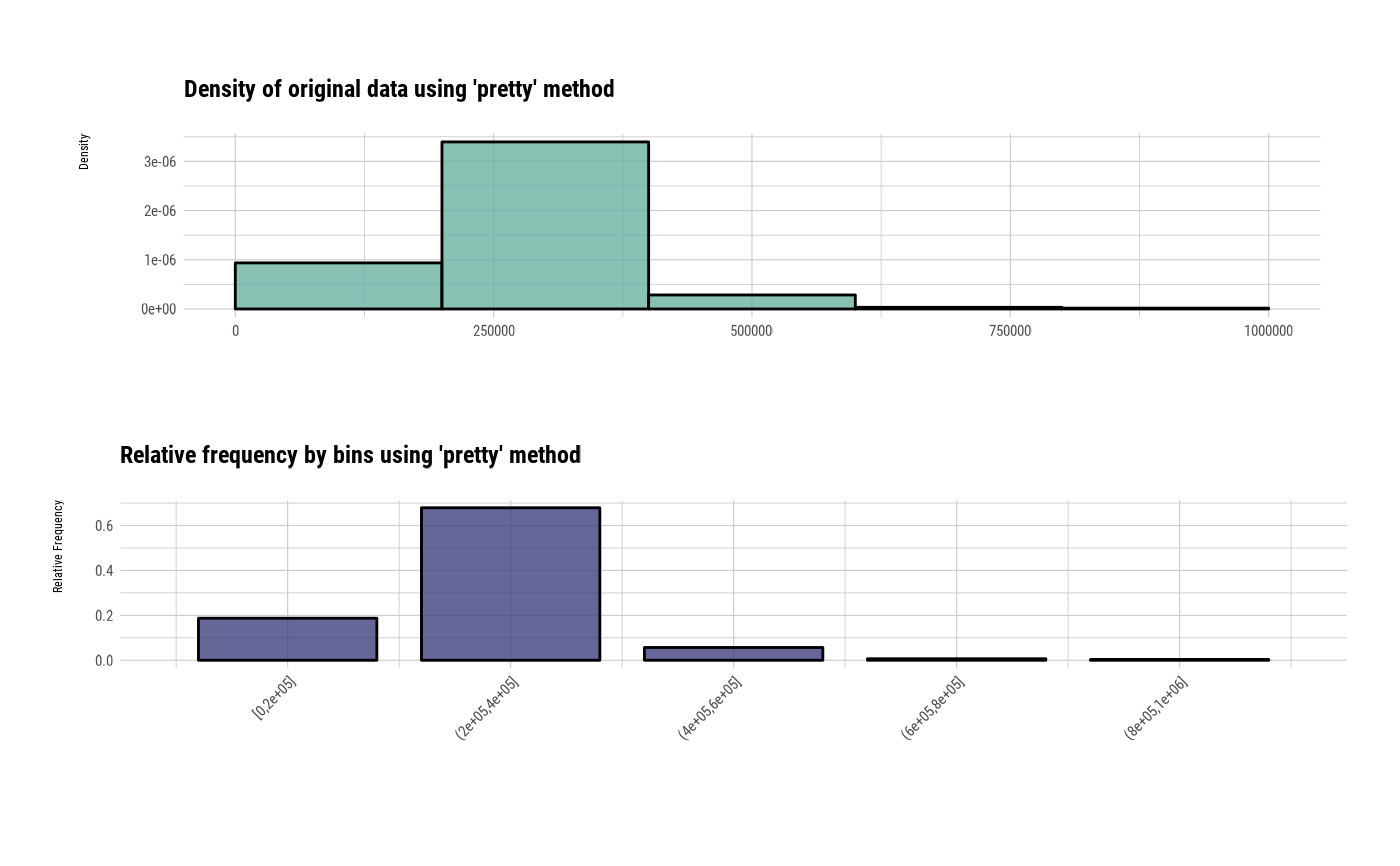bin <- binning(heartfailure2$platelets, nbins = 5, type = "kmeans")
plot(bin)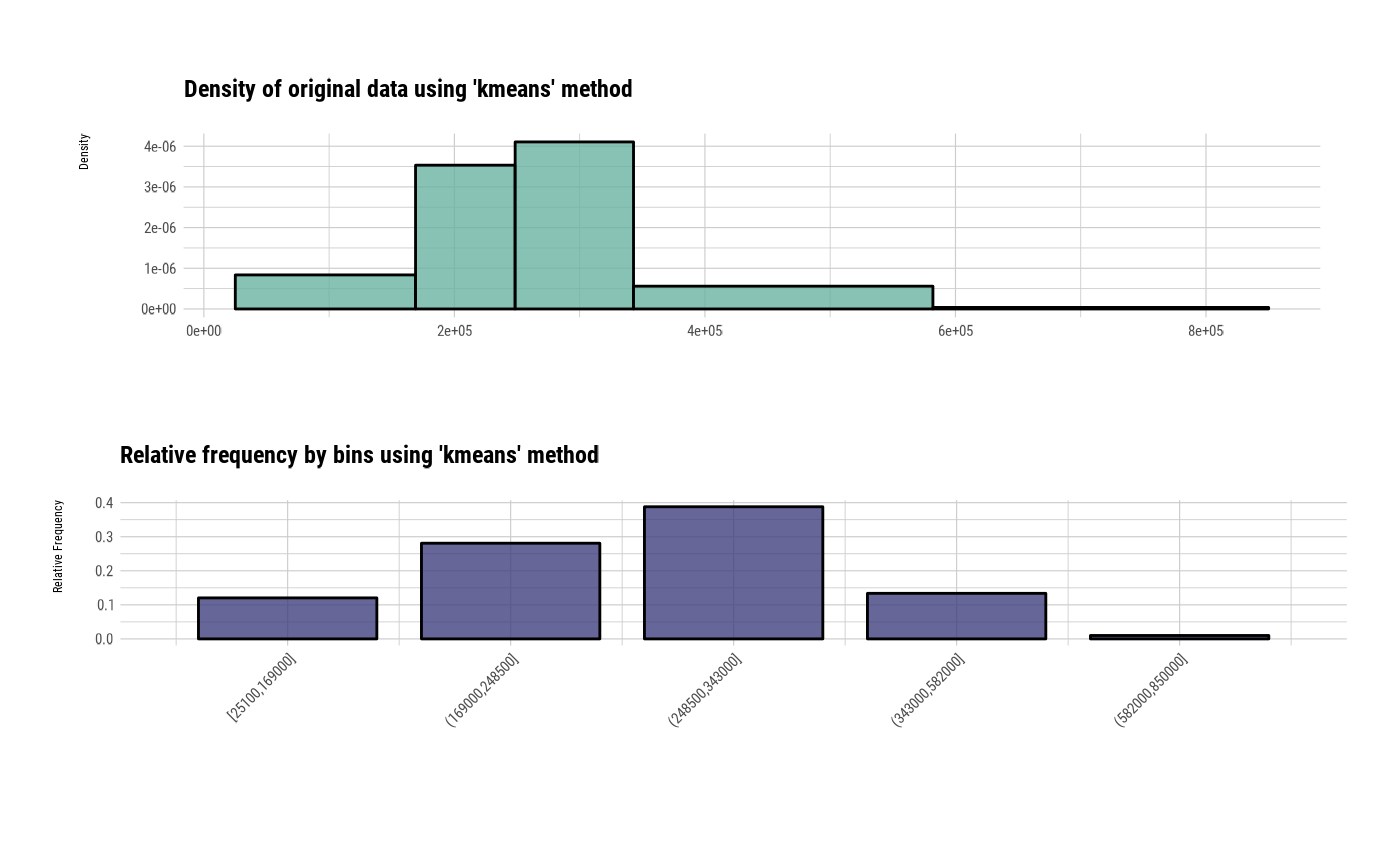bin <- binning(heartfailure2\$platelets, nbins = 5, type = "bclust")
plot(bin)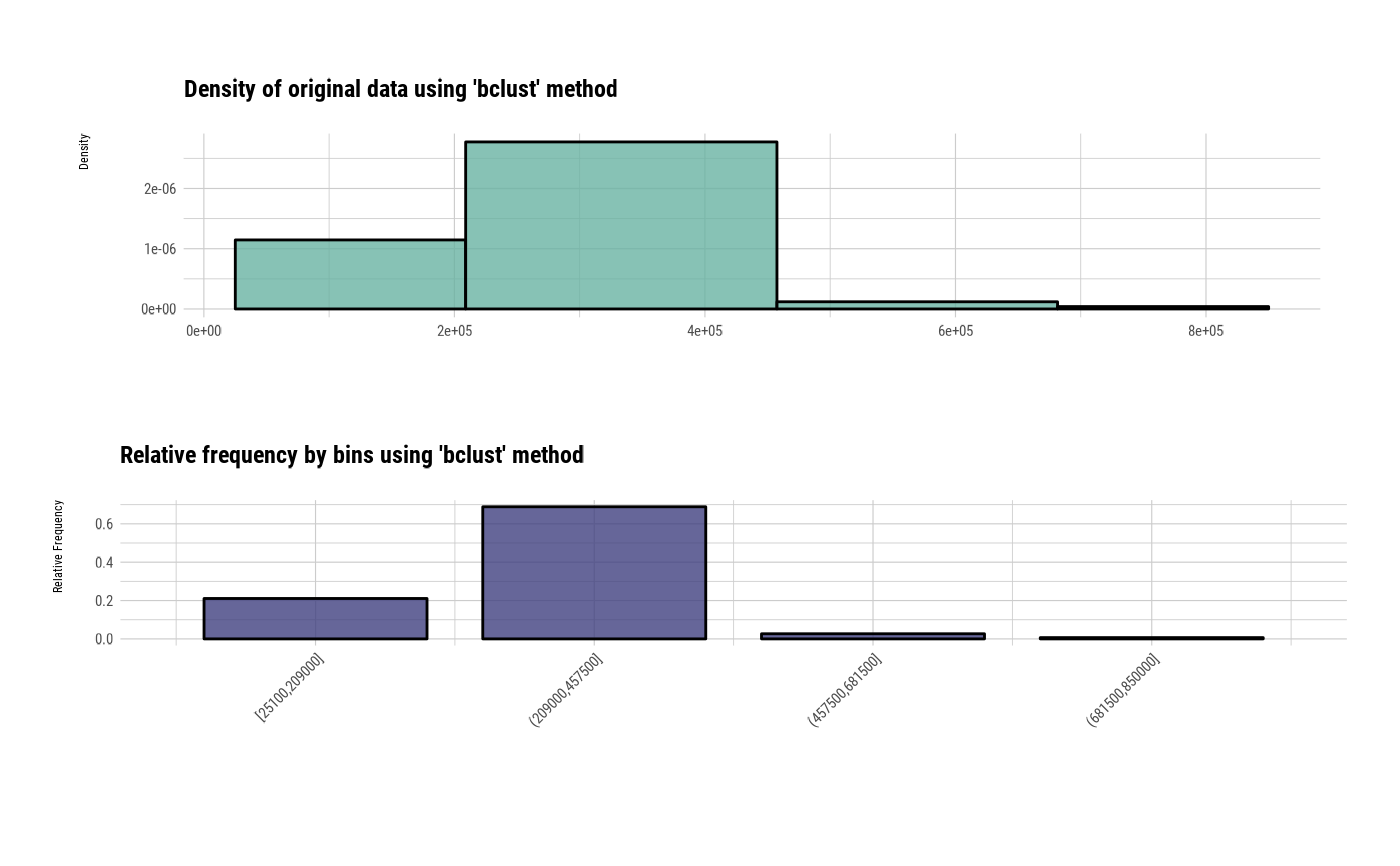# }